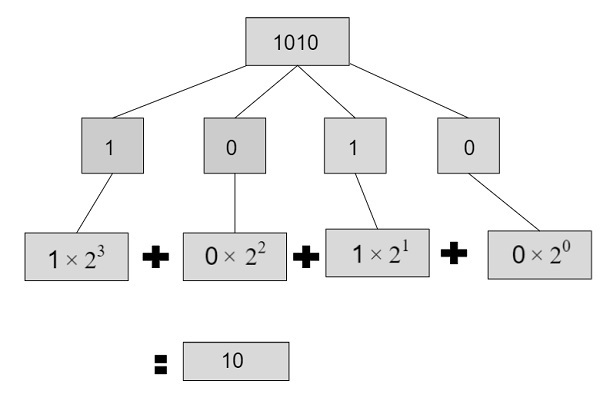# Program for Binary To Decimal Conversion in C++

Given with a binary number as an input, the task is to convert the given binary number into a decimal number.

Decimal number in computers is represented with base 10 and binary number is represented with base 2 as it has only two binary digits 0 and 1 whereas decimal numbers can be any numeric digit starting from 0 – 9.

To convert a binary number into a decimal number we will extract digits starting from right to left through a remainder and then multiply it with the power of 2 starting from 0 and will be increased by 1 till the (number of digits) – 1 and with that keep adding the multiplied values to obtain final decimal value.

Given below is the pictorial representation of converting a binary number into a decimal number.## Example

Input-: 1010
0 will be converted to a decimal number by -: 0 X 2^0 = 0
1 have corresponding binary equivalent of 3 digit -: 1 X 2^1 = 2
0 have corresponding binary equivalent of 3 digit -: 0 X 2^2 = 0
1 have corresponding binary equivalent of 3 digit -: 1 X 2^3 = 8
Output-: total = 0 + 2 + 0 + 8 = 10

## Algorithm

Start
Step1-> Declare function to convert binary to decimal
int convert(string str)
set string n = str
set int val = 0
set int temp = 1
set int len = n.length()
Loop For int i = len - 1 i >= 0 i—
IF n[i] == '1'
Set val += temp
End
Set temp = temp * 2
End
return val
Step 2-> In main()
Set string val = "11101"
Call convert(val)
Stop

## Example

Live Demo

#include <iostream>
#include <string>
using namespace std;
//convert binary to decimal
int convert(string str) {
string n = str;
int val = 0;
int temp = 1;
int len = n.length();
for (int i = len - 1; i >= 0; i--) {
if (n[i] == '1')
val += temp;
temp = temp * 2;
}
return val;
}
int main() {
string val = "11101";
cout<<val<<" after converion into deciaml : "<<convert(val);
}

## Output

IF WE RUN THE ABOVE CODE IT WILL GENERATE FOLLOWING OUTPUT

11101 after converion into deciaml : 29